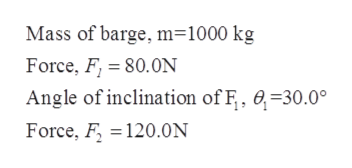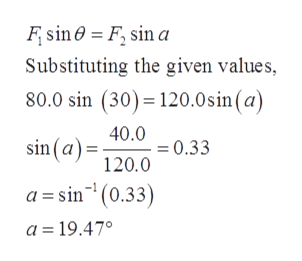# A 1000-kg barge is being towed by means of two horizontal cables. One cable is pulling with a force of 80.0 N in a direction of 30.0 degrees west of north. In What direction should the second cable pull so that the barge will accelerate northward, if the force exerted by the cable is 120 N? Assume that the water exerts no appreciable frictional drag on the barge.

Question
857 views

A 1000-kg barge is being towed by means of two horizontal cables. One cable is pulling with a force of 80.0 N in a direction of 30.0 degrees west of north. In What direction should the second cable pull so that the barge will accelerate northward, if the force exerted by the cable is 120 N? Assume that the water exerts no appreciable frictional drag on the barge.

check_circle

Step 1

The direction of the cable exerting force of 120 N can be calculated using following steps:

Given:help_outlineImage TranscriptioncloseMass of barge, m=1000 kg Force, F 80.0N Angle of inclination of F, 0-30.0° Force, F120.0N fullscreen
Step 2

Calculations:

The free body diagram of barge is given by,

Step 3

The equation of force acting on the ba...help_outlineImage TranscriptioncloseF sin a Fsin0 Substituting the given values, 80.0 sin (30) 120.0 sin (a) 40.0 0.33 sin(a)120.0 a sin(0.33 a 19.47° fullscreen

### Want to see the full answer?

See Solution

#### Want to see this answer and more?

Solutions are written by subject experts who are available 24/7. Questions are typically answered within 1 hour.*

See Solution
*Response times may vary by subject and question.
Tagged in

### Other